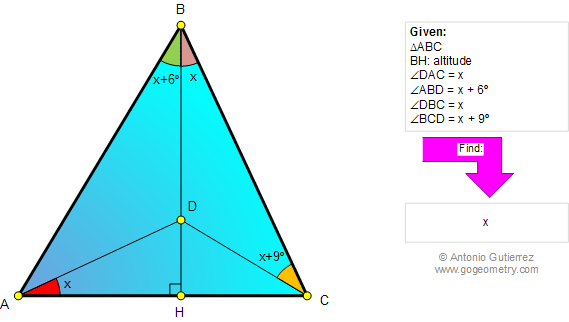# Online Geometry Problem 796: Triangle, Altitude, Measurement of Angles. Level: High School, SAT Prep, College, Mathematics Education

 The figure below shows a triangle ABC with a point D on the altitude BH. If angles DAC, ABD, DBC, and BCD are equal to x, x + 6, x, and x+9 degrees, respectively, find x.Home | Search | Geometry | Problems | All Problems | Open Problems | Visual Index | 10 Problems | 791-800 | Triangles | Altitude | Perpendicular | Angles | Email | Solution / comment | By Antonio Gutierrez